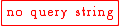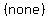# Find the number of permutations of the first eight letters of the alphabet taking five letters at a

User Generated

JubNzV

Mathematics

### Question Description

Time. A.1680 B.56 C.6720 D.109Student has agreed that all tutoring, explanations, and answers provided by the tutor will be used to help in the learning process and in accordance with Studypool's honor code & terms of service.Since order does matter (this is because the word "cab" is different than "abc"), we can use the permutation formulaStart with the given formulaPlug in(this is number of letters given) and(this is the number of letters we can use together at a time)Subtractto get 5

Expand 8!Expand 5!CancelSimplifyNow multiply 8*7*6 to get 336

So 8 choose 3 (where order does matter) yields 336 unique combinationsfvzfvcyr (622)
New York UniversityAnonymous
Great study resource, helped me a lot.Studypool4.7Trustpilot4.5Sitejabber4.4